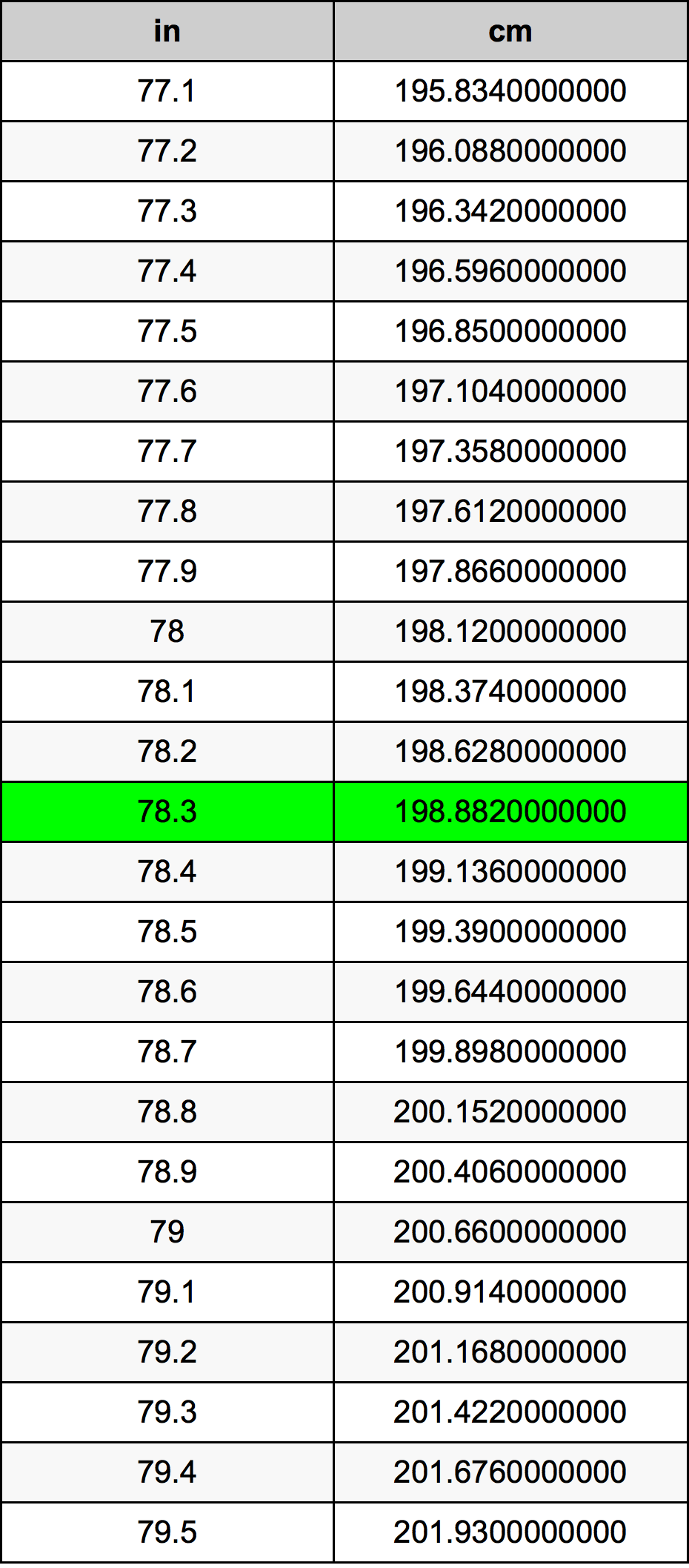Inches To Centimeters

# 78.3 in to cm78.3 Inches to Centimeters

in
=
cm

## How to convert 78.3 inches to centimeters?

 78.3 in * 2.54 cm = 198.882 cm 1 in
A common question is How many inch in 78.3 centimeter? And the answer is 30.8267716535 in in 78.3 cm. Likewise the question how many centimeter in 78.3 inch has the answer of 198.882 cm in 78.3 in.

## How much are 78.3 inches in centimeters?

78.3 inches equal 198.882 centimeters (78.3in = 198.882cm). Converting 78.3 in to cm is easy. Simply use our calculator above, or apply the formula to change the length 78.3 in to cm.

## Convert 78.3 in to common lengths

UnitUnit of length
Nanometer1988820000.0 nm
Micrometer1988820.0 µm
Millimeter1988.82 mm
Centimeter198.882 cm
Inch78.3 in
Foot6.525 ft
Yard2.175 yd
Meter1.98882 m
Kilometer0.00198882 km
Mile0.0012357955 mi
Nautical mile0.0010738769 nmi

## What is 78.3 inches in cm?

To convert 78.3 in to cm multiply the length in inches by 2.54. The 78.3 in in cm formula is [cm] = 78.3 * 2.54. Thus, for 78.3 inches in centimeter we get 198.882 cm.

## 78.3 Inch Conversion Table## Alternative spelling

78.3 in to cm, 78.3 in in cm, 78.3 Inches to Centimeters, 78.3 Inches in Centimeters, 78.3 Inch to Centimeter, 78.3 Inch in Centimeter, 78.3 in to Centimeters, 78.3 in in Centimeters, 78.3 Inches to cm, 78.3 Inches in cm, 78.3 Inch to cm, 78.3 Inch in cm, 78.3 Inch to Centimeters, 78.3 Inch in Centimeters